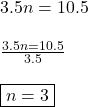## Explain how to solve the equation 3.5n = 10.5 , what is the value of n ?

Question

Explain how to solve the equation 3.5n = 10.5 , what is the value of n ?

in progress 0
4 weeks 2021-08-19T13:23:03+00:00 2 Answers 0 views 0

n=3

Step-by-step explanation:

Divide both sides by 3.5

n=  3.5
/10.5

Simplify  3.5
/10.5  to  3.

N=3